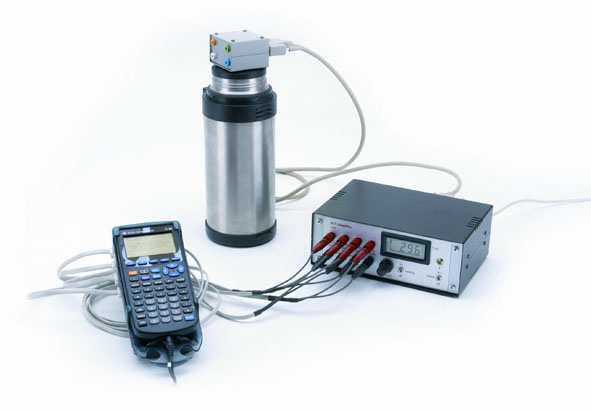Measurement of resistance vs. temperature for various materialsThe apparatus allows to investigate the temperature dependance of resistivity in metals and semiconductors, from T=80K (liquid nitrogen) to T=450K (about 180 C), using a real-time data acquisition system based either on  Personal Computer or on handheld Graphing Calculators . By measuring simultaneously the signals related to three different samples while temperature is changing, one may compare different behaviors: the quasi-linear one in metals (where the carriers density is constant and mainly phonon scattering modulates the electron mobility), and the low temperature increase followed by exponential drop at high temperature in doped semiconductors (where electron-hole pairs are generated in the intrinsic region). The value Eg(0) of the semiconductor energy gap extrapolated to T=0K may be obtained through a simple data analysis. see: An undergraduate laboratory experiment for measuring the energy gap in semiconductors,  Eur.J.Phys..19,123 (1989)